#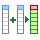Unite

Function paste several columns (variables) into one.

## Properties

 Variables to Unite Selection of dataset variables (columns) that you want to combine into one variable. Col Name The name of new column (variable). Separator Character. String used as separator between values from individual variables. Remove Input Boolean. Property defines whether combined variables from the input table will be removed. Ignore NA Boolean. Property determines if blank cells will be ignored (skipped).

## Keywords

combine columns, combine cells, multiple columns into one, merge excel columns, merce cells, stack columns, combine multiple columns, CONCATENATE, concatenate alternatives, join excel columns, unite columns

## How to Combine Several Excel Columns into One

Often, we need to combine multiple variables into one. In Excel we use the CONCATENATE function for this purpose. The alternative inside the Reshape.XL add-in is Unite function. This feature contains several adjustable parameters that make it more efficient. As an example I can mention the work with empty cells. An example is shown in the following figure.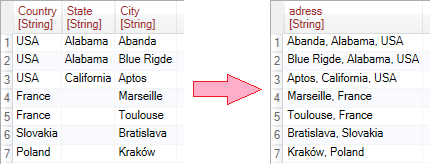In this tutorial, we will use a relatively simple input table, an example of which is shown in the following figure. This table contains three variables - Country, State and City. Our task will be to combine these variables into one.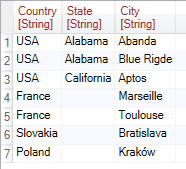For the purpose of combining multiple variables into one, we use the Unite function from the Variables ribbon toolbar tab. Clicking this button will display the function in the sidebar. In the Properties panel you can find adjustable parameters. These settings are displayed in two tabs - Variables and Properties.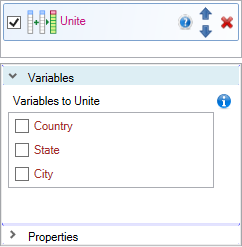In the Variables tab, you select variables (table columns) that you want to combine together. You make their choice using a check-boxes. After variable selection, an integer index is displayed at the end of the row, which determines the order in which variables will be combined.

In the following example, we combined the variables in the order of Country, State and City. Function created a table with one variable - Address, which contains the values of the selected variables (columns). The default value “_” was used as a separator between values. Finally, the variables used for merging were automatically deleted from the table. Also as you can see, empty cells from the State variable (the last 4 lines) were ignored when joining.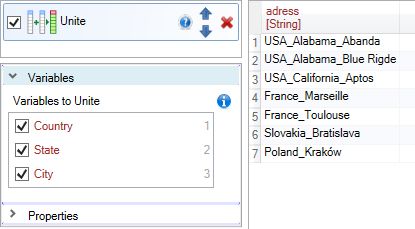If you select variables in a different order, this order is reflected in the resulting created variable. In the following example, we combined the variables in the reverse order - City, State and Country.In the Properties tab, you can set auxiliary parameters. In the first row (Col Name) you can set the name of the resulting variable.

By using a second property - Separator, you can define a text string to be applied between the values of the individual variables. In the following example, we used a comma. The default value is underscore.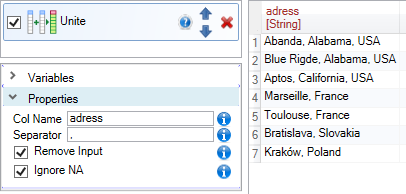With the boolean property Remove Input, you can set whether the combined variables in the result table will be deleted. By default, the property is set to TRUE. The following example shows the change to FALSE. In this case the input variables were left in the table.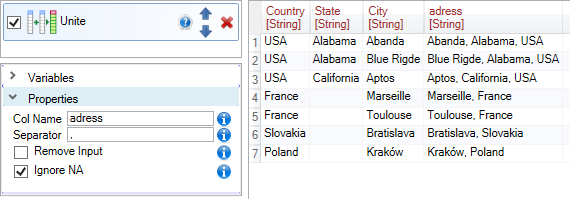Finally, you have the Ignore NA property. The Unite function automatically recognizes empty cells. If the Ignore NA property is set to TRUE, the empty cell is ignored. If set to FALSE, empty cells will be used in the result. This means that if the cell is empty, the resulting value of the combined variables include twice repeating of string defined through the Separator property. In the following example, you can see the string “, , “ in the last four rows in the Address variable.Using the Unite function is very easy and fast. On the other hand, if you want to divide one variable (table column) into several new variables, you can use Separate function. As an alternative to the Separate function, you can use also the Separate_To_Rows function. This function splits one cell into several table rows.